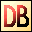DelphiBasicsSinFunction The Sine of a number System unit
 function Sin ( const Number : Extended ) : Extended;
Description
The Sin function is a mathematical function giving the Sine value of a Number value given radians.

 PI radians = 180 degrees
Notes
The System unit supports only the following angular functions:

ArcTan
Sin
Cos

Related commands
 ArcCos The Arc Cosine of a number, returned in radians ArcSin The Arc Sine of a number, returned in radians ArcTan The Arc Tangent of a number, returned in radians Cos The Cosine of a number Tan The Tangent of a number

Buy Website Traffic atDownload this web site as a Windows program.